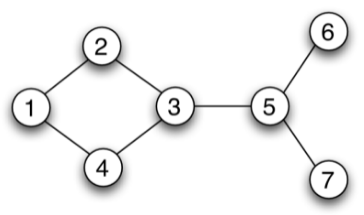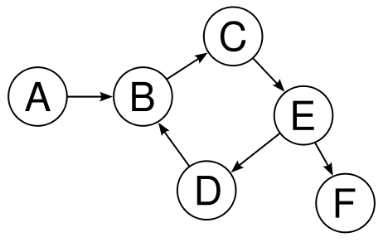# Undirected and Directed Graph Properties

Today we are back and talking about the properties of undirected graphical models and directed graphical models. The term undirected simply means that the edges connecting the nodes of the graph do not flow in a particular direction. Conversely, directed graphs have edges that only allow flow in certain directions.

## Undirected Graphs and Global Markov Properties

Let’s say we have an undirected graph (UG) written as $$G = (V, E)$$ where the nodes, $$V$$, represent variables in a random vector of variables, $$X$$. There are some properties that the random vector, $$X$$, can satisfy to ensure conditional independence. We call these “global Markov properties” and we should be able to find them just by looking at the graph structure .Figure 1 - Source 

Let’s consider a UG as shown in Figure 1. We can say there is a path from node 1 to 6 that runs as follows: 1 -> 2 -> 3 -> 5 -> 6. This is an active path given nodes {4, 7} because nodes 4 and 7 are not contained in this path .

Conversely, if we want to write down what nodes separate node 1 from node 6, then we would say that the set Z = {2, 3, 5} separates nodes 1 and 6. This is because there is no active path from 1 to 6 without these nodes. In other words, if we remove the nodes 2, 3 and 5, then nodes 1 and 6 lie in disconnected graph components. This idea of separation for UGs can be written as :

$\text{SEP}_G(X, Y | Z)$

More formally then, for a given graph G, I can write a list of conditional independencies like so :

$\mathcal{I}(G) = {X \perp \!\!\! \perp Y | Z : \text{SEP}_G(X, Y | Z)}$

This list is called the global Markov properties of the UG $$G$$ .

There is an interesting idea in graphical models that there is an overarching distribution, $$P$$, which can be represented by some graph $$G$$. We often say that the distribution $$P$$ factors according to $$G$$. We can also say that any distribution $$P$$ that factors according to $$G$$ will satisfy the global Markov properties associated with $$G$$, that is $$\mathcal{I}(G) \subseteq \mathcal{I}(P)$$, where $$\mathcal{I}(P)$$ is the set of all conditional independencies satisfied by $$P$$ .

We also have 2 other kinds of Markov properties for a UG. First, there exist pairwise Markov properties which is the set :

$\mathcal{I}_p(G) = {X \perp \!\!\! \perp Y | \mathcal{X} - {X, Y} : (X, Y) \notin E(G)}$

Where $$\mathcal{X} = X \cup Y \cup Z$$. This basically says that $$X$$ and $$Y$$ are conditionally independent given all the nodes that are not contained in $$X$$ and $$Y$$, where there are no edges directly connecting $$X$$ and $$Y$$ (i.e. where they are disconnected subgraphs given all the other nodes in the graph) .

In order to introduce the other kind of Markov properties, we first need to define a Markov blanket as follows :

$MB_G(X) = \text{NBRS}(X)$

Where $$\text{NBRS}(X)$$ means the set of neighbors of nodes X. So now I can describe the local Markov properties where :

$\mathcal{I}_l(G) = {X \perp \!\!\! \perp \mathcal{X} - {X} - MB_G(X) | MB_G(X)}$

This means that the nodes in X are conditionally independent of all the other nodes in the graph except other nodes in X and the immediate neighbors of X, given the neighbors of X. That is, if we removed all the immediate neighbors of X from the graph, that would make X a disconnected graph from the rest of $$\mathcal{X}$$, indicating that it is conditionally independent from everything else in the graph .

## D-Separation for DGMs

Similar to UGMs, for DGMs we can say that two sets of nodes, $$X$$ and $$Y$$, are conditionally independent given another set of nodes, $$Z$$, if the nodes $$Z$$ separate $$X$$ and $$Y$$. However, the idea of separation for DGMs is a little more complicated than for UGMs, so we need to spend some time defining the DGM-specific form of separation, $$\text{DSEP}_G$$. So far, we know that :

$\mathcal{I}(G) = {X \perp \!\!\! \perp Y | Z : \text{DSEP}_G(X, Y | Z)}$

Consider Figure 2. We say that there is a path from A to F as long as there is a set of edges connecting them (not necessarily all pointing the same way). For example, a valid path from A to F would be A -> B -> C -> E -> F. We would say that A was d-separated from F if all the paths from A to F were blocked by some subset $$Z$$, which in this case might be $$Z = {B, C, D, E}$$ .Figure 2 - Source 

On the surface this might seem as straightforward as the notion of separation for UGMs. But there are some cases where this will get more complicated. Let’s look at some of them :

• Causal trail: $$X \rightarrow Z \rightarrow Y$$ is blocked (i.e. $$X \perp \!\!\! \perp Y$$) iff $$Z$$ is observed (i.e. given R$$Z$$).

• Evidential trail: $$X \leftarrow Z \leftarrow Y$$ is blocked iff $$Z$$ is observed.

• Common cause: $$X \leftarrow Z \rightarrow Y$$ is blocked iff Z is observed. One example of this is if X was shoe size and Y was gray hair. They are marginally dependent, but if we conditioned them on age, Z, then they are conditionally independent of each other now that we know how old the person is.

• Common effect: $$X \rightarrow Z \leftarrow Y$$ is blocked iff neither Z nor any of its descendents, $$\text{DESC}_Z$$ is observed. This indicates that $$\text{DSEP}(X, Y)$$ but not necessarily given $$Z$$. One way to think about this is if $$X$$ is vomiting and $$Y$$ is having a sore throat, and $$Z$$ is having a cold. If we know that we have a cold and a sore throat, then it is less likely that we are also vomiting, but it is not zero probability. That is, $$X$$ is not independent of $$Y$$ given $$Z$$. (In other words, I could be hungover and have a cold at the same time.)

Given what we know now, a path in a DGM is blocked given some set of nodes, $$Z$$, iff one of these two things are true :

1. There is a v-structure of consecutive nodes $$X_{i-1} \rightarrow X_i \leftarrow X_{i+1}$$ such that neither $$X_i$$ nor one of its descendents are in Z.

2. There is a node in $$Z$$ that is not a common child in a v-structure (i.e. that is not $$X_i$$).

 Ravikumar, P. “UGMs: Markov Properties.” 10-708: Probabilistic Graphical Models. 2021. Class notes.

 Terelius, Håkan. (2010). Distributed Multi-Agent Optimization via Dual Decomposition.

 Ravikumar, P. “DGMs: Markov Properties.” 10-708: Probabilistic Graphical Models. 2021. Class notes.

 “The web as a directed graph.” Computer Science Wiki. https://computersciencewiki.org/index.php/The_web_as_a_directed_graph Accessed 20 Sept 2021.